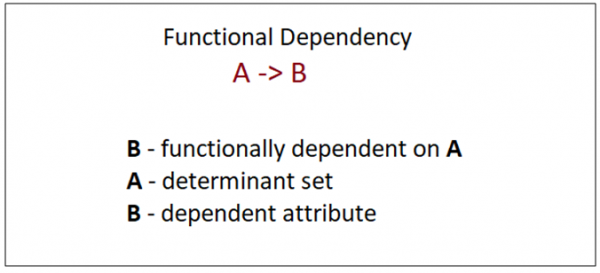# Functional dependency in DBMS

## What is Functional Dependency

Functional dependency in DBMS, as the name suggests is a relationship between attributes of a table dependent on each other. Introduced by E. F. Codd, it helps in preventing data redundancy and gets to know about bad designs.

To understand the concept thoroughly, let us consider P is a relation with attributes A and B. Functional Dependency is represented by -> (arrow sign)

Then the following will represent the functional dependency between attributes with an arrow sign −

 A -> B

Above suggests the following:## Example

The following is an example that would make it easier to understand functional dependency −

We have a <Department> table with two attributes − DeptId and DeptName.

 DeptId = Department IDDeptName = Department Name

The DeptId is our primary key. Here, DeptId uniquely identifies the DeptName attribute. This is because if you want to know the department name, then at first you need to have the DeptId.

 DeptId DeptName 001 Finance 002 Marketing 003 HR

Therefore, the above functional dependency between DeptId and DeptName can be determined as DeptId is functionally dependent on DeptName

 DeptId -> DeptName

## Types of Functional Dependency

Functional Dependency has three forms −

• Trivial Functional Dependency
• Non-Trivial Functional Dependency
• Completely Non-Trivial Functional Dependency

Let us begin with Trivial Functional Dependency −

## Trivial Functional Dependency

It occurs when B is a subset of A in −

 A ->B

Example

We are considering the same <Department> table with two attributes to understand the concept of trivial dependency.

The following is a trivial functional dependency since DeptId is a subset of DeptId and DeptName

 { DeptId,  DeptName } -> Dept Id

## Non –Trivial Functional Dependency

It occurs when B is not a subset of A in −

 A ->B

Example

 DeptId ->  DeptName

The above is a non-trivial functional dependency since DeptName is a not a subset of DeptId.

## Completely Non - Trivial Functional Dependency

It occurs when A intersection B is null in −

 A ->B

## Armstrong’s Axioms Property of Functional Dependency

Armstrong’s Axioms property was developed by William Armstrong in 1974 to reason about functional dependencies.

The property suggests rules that hold true if the following are satisfied:

• TransitivityIf A->B and B->C, then A->C i.e. a transitive relation.
• ReflexivityA-> B, if B is a subset of A.
• AugmentationThe last rule suggests: AC->BC, if A->B

Updated on: 13-Sep-2023

25K+ Views